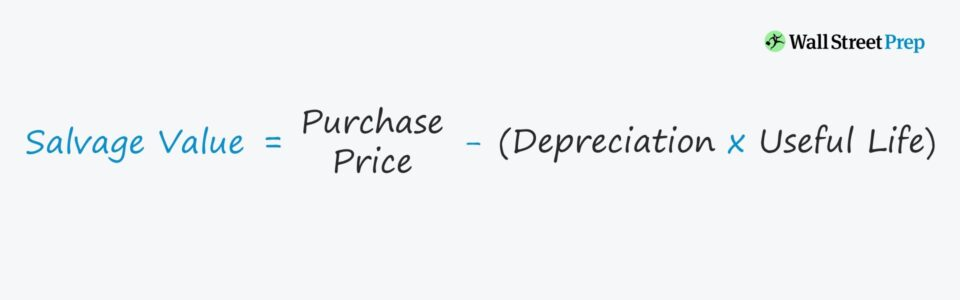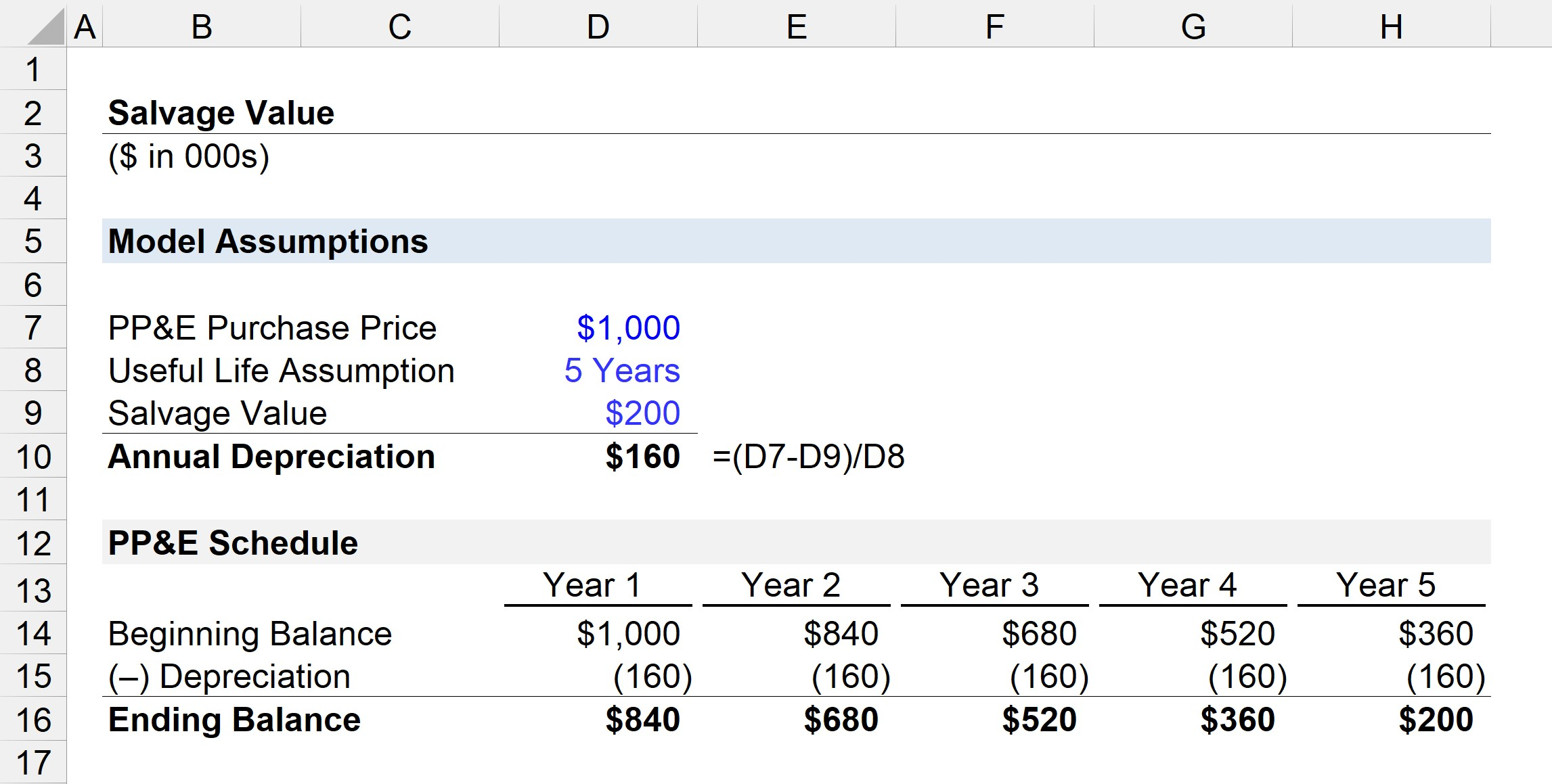Welcome to Wall Street Prep! Use code at checkout for 15% off.# Salvage Value

Guide to Understanding Salvage Value## How to Calculate Salvage Value?

The salvage value is considered the resale price of an asset at the end of its useful life.

In order words, the salvage value is the remaining value of a fixed asset at the end of its useful life.

Under accrual accounting, the cost of purchasing PP&E like machinery and equipment – i.e. capital expenditures (Capex) – is expensed on the income statement and spread out across the useful life assumption.

The useful life assumption estimates the number of years an asset is expected to remain productive and generate revenue.

The carrying value of the asset is then reduced by depreciation each year during the useful life assumption.

Calculating the residual value of the fixed asset is a three-step process:

1. Determine Purchase Price → The original cost incurred to acquire the fixed asset (PP&E).
2. Calculate Total Depreciation → The annual depreciation expense is multiplied by the useful life assumption (or number of years in which the fixed asset was depreciated).
3. Subtract Cumulative Depreciation from Purchase Price → The original purchase price is deducted by the cumulative depreciation expensed across the assumed useful life.

## Salvage Value Formula

The formula to calculate the salvage value is as follows.

Salvage Value = Purchase Price(Annual Depreciation × Number of Years)

Starting from the original cost of purchase, we must deduct the product of the annual depreciation expense and the number of years.

## Salvage Value Depreciation Equation

The calculate the annual depreciation expense, three inputs are necessary:

1. Purchase Price of Asset
2. Salvage (Residual) Value
3. Useful Life Assumption

The difference between the asset purchase price and the salvage (residual) value is the total depreciable amount.

To calculate the annual depreciation expense, the depreciable cost (i.e. the asset’s purchase price minus the residual value assumption) is divided by the useful life assumption.

Annual Depreciation = (Purchase PriceSalvage Value)÷ Useful Life

If the residual value assumption is set as zero, then the depreciation expense each year will be higher, and the tax benefits from depreciation will be fully maximized.

The impact of the salvage (residual) value assumption on the annual depreciation of the asset is as follows.

• Higher Salvage Value → Lower Annual Depreciation
• Lower Salvage Value → Higher Annual Depreciation

Under straight-line depreciation, the asset’s value is reduced in equal increments per year until reaching a residual value of zero by the end of its useful life.

The majority of companies assume the residual value of an asset at the end of its useful life is zero, which maximizes the depreciation expense (and tax benefits).

## Car Salvage Value Calculation Example

Briefly, suppose we’re currently attempting to determine the salvage value of a car, which was purchased four years ago for \$100,000.

We’ll assume the useful life of the car is ten years, at which the car is practically worthless by then, i.e. for the sake of simplicity, we’ll assume the scrap value is zero by the end of its useful life.

• Purchase Price = \$100,000
• Scrap Value = \$0
• Useful Life = 10 Years
• Number of Years Passed = 4 Years

Q. If you’re attempting to sell the used car to your friend, how much should you expect?

Each year, the depreciation expense is \$10,000 and four years have passed, so the accumulated depreciation to date is \$40,000.

• Depreciation Expense = \$100,000 ÷ 10 Years = \$10,000
• Accumulated Depreciation = \$10,000 × 4 Years = \$40,000

There are six years remaining in the car’s total useful life, thus the estimated price of the car should be around \$60,000.

• Estimated Resale Value = \$100,000 – \$40,000 = \$60,000

But of course, there are other variables to consider to be realistic, i.e. the straight-line methodology we utilized here is NOT appropriate for peer-to-peer transactions because a substantial portion of the car’s depreciation occurs in the earlier years.

Hence, a car with even a couple of miles driven on it tends to lose a significant percentage of its initial value the moment it becomes a “used” car.

## Salvage Value Calculator

We’ll now move to a modeling exercise, which you can access by filling out the form below.#### Get the Excel Template!Submitting...

## Fixed Asset Salvage Value Calculation Example (PP&E)

Suppose a company spent \$1 million purchasing machinery and tools, which are expected to be useful for five years and then be sold for \$200k.

Given the information above, the model assumptions are as follows.

• PP&E Purchase Price = \$1 million
• Useful Life Assumption = 5 Years
• Salvage Value = \$200k

Next, the annual depreciation can be calculated by subtracting the residual value from the PP&E purchase price and dividing that amount by the useful life assumption.

• Annual Depreciation = (\$1 million – \$200k) ÷ 5 Years = \$160k

While the purpose of this exercise is to see how our salvage (residual) value assumption impacts the annual depreciation expense, which in turn impacts the PP&E balance – we can also calculate the residual asset value using the formula discussed earlier.

• Salvage Value = \$1 million – (\$160k × 5 Years) = \$200k

The beginning balance of the PP&E is \$1 million in Year 1, which is subsequently reduced by \$160k each period until the end of Year 5.

By the end of the PP&E’s useful life, the ending balance should be equal to our \$200k assumption – which our PP&E schedule below confirms.Step-by-Step Online Course

### Everything You Need To Master Financial Modeling

Enroll in The Premium Package: Learn Financial Statement Modeling, DCF, M&A, LBO and Comps. The same training program used at top investment banks.

Inline Feedbacks